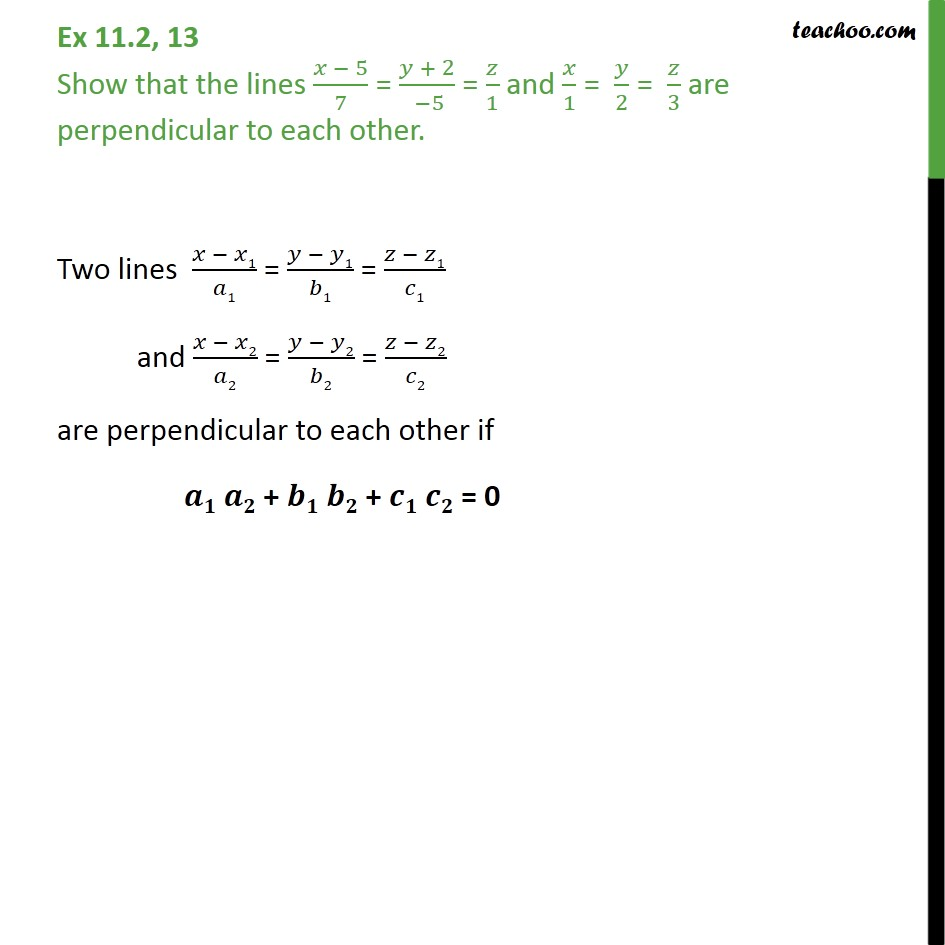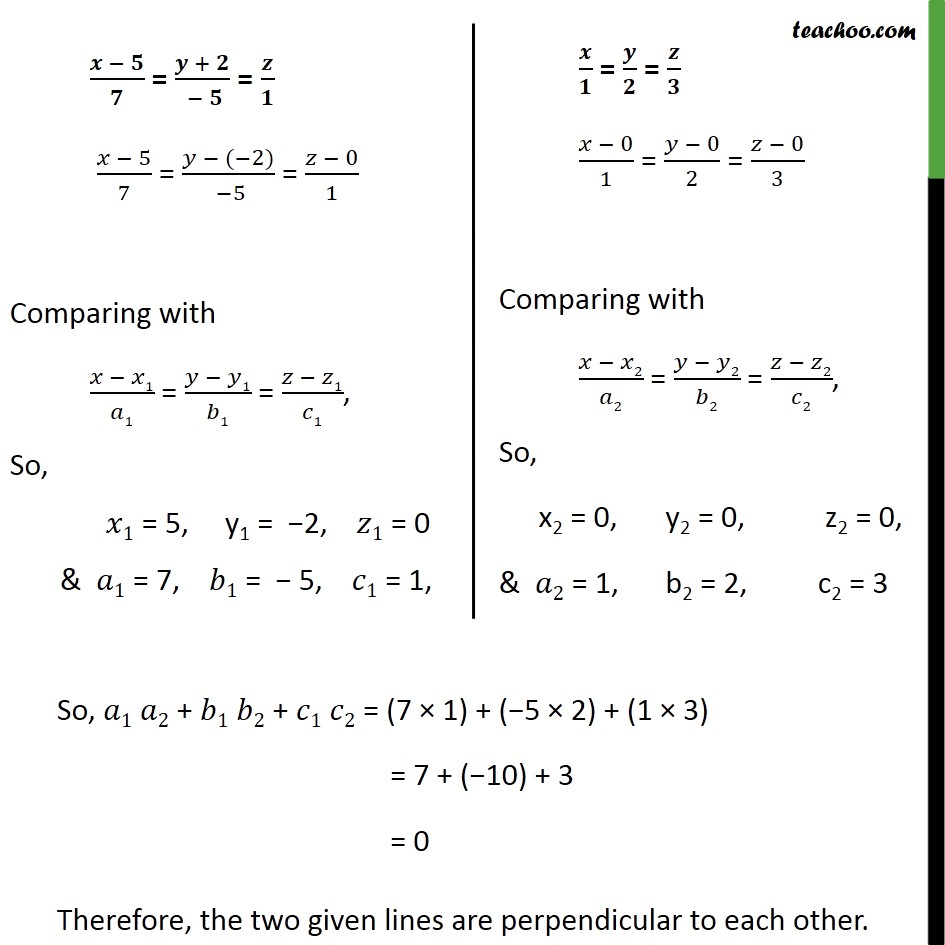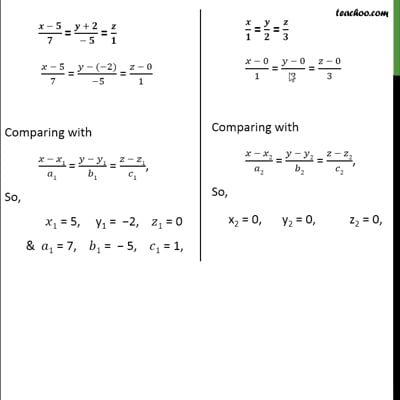Ex 11.2

Chapter 11 Class 12 Three Dimensional Geometry
Serial order wiseThis video is only available for Teachoo black users

Solve all your doubts with Teachoo Black (new monthly pack available now!)

### Transcript

Ex 11.2, 13 Show that the lines 5 7 = + 2 5 = 1 and 1 = 2 = 3 are perpendicular to each other. Two lines 1 1 = 1 1 = 1 1 and 2 2 = 2 2 = 2 2 are perpendicular to each other if + + = 0 So, 1 2 + 1 2 + 1 2 = (7 1) + ( 5 2) + (1 3) = 7 + ( 10) + 3 = 0 Therefore, the two given lines are perpendicular to each other.